x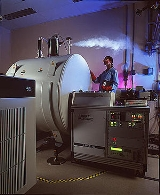Fourier transform ion cyclotron resonanceEncyclopedia
Fourier transform ion cyclotron resonance mass spectrometry, also known as Fourier transform mass spectrometry, is a type of mass analyzer (or mass spectrometer) for determining the mass-to-charge ratio
Mass-to-charge ratio
The mass-to-charge ratio ratio is a physical quantity that is widely used in the electrodynamics of charged particles, e.g. in electron optics and ion optics. It appears in the scientific fields of lithography, electron microscopy, cathode ray tubes, accelerator physics, nuclear physics, Auger...

(m/z) of ions based on the cyclotron frequency
Ion cyclotron resonance
Ion cyclotron resonance is a phenomenon related to the movement of ions in a magnetic field. It is used for accelerating ions in a cyclotron, and for measuring the masses of an ionized analyte in mass spectrometry, particularly with Fourier transform ion cyclotron resonance mass spectrometers...

of the ions in a fixed magnetic field. The ions are trapped in a Penning trap
Penning trap
Penning traps are devices for the storage of charged particles using a homogeneous static magnetic field and a spatially inhomogeneous static electric field. This kind of trap is particularly well suited to precision measurements of properties of ions and stable subatomic particles which have...

(a magnetic field with electric trapping plates) where they are excited to a larger cyclotron radius by an oscillating electric field perpendicular to the magnetic field. The excitation also results in the ions moving in phase (in a packet). The signal is detected as an image current on a pair of plates which the packet of ions passes close to as they cyclotron. The resulting signal is called a free induction decay
Free induction decay
In Fourier Transform NMR, free induction decay is the observable NMR signal generated by non-equilibrium nuclear spin magnetisation precessing about the magnetic field ....

(FID), transient or interferogram that consists of a superposition of sine waves. The useful signal is extracted from this data by performing a Fourier transform
Fourier transform
In mathematics, Fourier analysis is a subject area which grew from the study of Fourier series. The subject began with the study of the way general functions may be represented by sums of simpler trigonometric functions...

to give a mass spectrum
Mass spectrum
A mass spectrum is an intensity vs. m/z plot representing a chemical analysis. Hence, the mass spectrum of a sample is a pattern representing the distribution of ions by mass in a sample. It is a histogram usually acquired using an instrument called a mass spectrometer...

.

Fourier transform ion cyclotron resonance (FTICR) mass spectrometry is a very high resolution technique in that masses can be determined with very high accuracy. Many applications of FTICR-MS use this mass accuracy to help determine the composition of molecules based on accurate mass. This is possible due to the mass defect of the elements. FT-ICR MS is able to achieve higher levels of resolution than other forms of mass spectrometry, in part, because a superconducting magnet is much more stable than rf voltage. Another place that FTICR-MS is useful is in dealing with complex mixtures since the resolution (narrow peak width) allows the signals of two ions of similar mass to charge (m/z) to be detected as distinct ions. This high resolution is also useful in studying large macromolecules such as proteins with multiple charges which can be produced by electrospray ionization
Electrospray ionization
Electrospray ionization is a technique used in mass spectrometry to produce ions. It is especially useful in producing ions from macromolecules because it overcomes the propensity of these molecules to fragment when ionized...

. For example, attomole level of detection of two peptides has been reported . These large molecules contain a distribution of isotopes that produce a series of isotopic peaks. Because the isotopic peaks are close to each other on the m/z axis, due to the multiple charges, the high resolving power of the FTICR is extremely useful.

FTICR-MS differs significantly from other mass spectrometry
Mass spectrometry
Mass spectrometry is an analytical technique that measures the mass-to-charge ratio of charged particles.It is used for determining masses of particles, for determining the elemental composition of a sample or molecule, and for elucidating the chemical structures of molecules, such as peptides and...

techniques in that the ions are not detected by hitting a detector such as an electron multiplier
Electron multiplier
An electron multiplier is a vacuum-tube structure that multiplies incident charges. In a process called secondary emission, a single electron can, when bombarded on secondary emissive material, induce emission of roughly 1 to 3 electrons...

but only by passing near detection plates. Additionally the masses are not resolved in space or time as with other techniques but only by the cyclotron (rotational) frequency that each ion produces as it rotates in a magnetic field. Thus, the different ions are not detected in different places as with sector instrument
Sector instrument
A sector instrument is a general term for a class of mass spectrometer that uses a static electric or magnetic sector or some combination of the two as a mass analyzer. A popular combination of these sectors has been the BEB...

s or at different times as with time-of-flight
Time-of-flight
Time of flight describes a variety of methods that measure the time that it takes for an object, particle or acoustic, electromagnetic or other wave to travel a distance through a medium...

instruments but all ions are detected simultaneously over some given period of time. In FT-ICR MS, resolution can be improved by increasing the strength of the magnet (in teslas) or by increasing the detection duration.

## History

FT-ICR was invented by Melvin B. Comisarow and Alan G. Marshall
Alan G. Marshall
Alan G. Marshall is an American analytical chemist who has devoted his scientific career to developing a scientific technique known as Fourier transform ion cyclotron resonance mass spectrometry, which he co-invented. He was born in Bluffton, Ohio, in 1944, and earned his Bachelor's in Chemistry...

at the University of British Columbia
University of British Columbia
The University of British Columbia is a public research university. UBC’s two main campuses are situated in Vancouver and in Kelowna in the Okanagan Valley...

. The first paper appeared in Chemical Physics Letters
Chemical Physics Letters
Chemical Physics Letters is a peer-reviewed scientific journal, published since 1967 by Elsevier. It publishes brief papers in the field of Chemical Physics. The current editors are David Clary, Villy Sundström and the 1999 Nobel Prize in Chemistry winner, Ahmed Zewail....

in 1974. The inspiration was earlier developments in conventional ICR and Fourier Transform Nuclear Magnetic Resonance (FT-NMR) spectroscopy. Marshall has continued to develop the technique at The Ohio State University
Ohio State University
The Ohio State University, commonly referred to as Ohio State, is a public research university located in Columbus, Ohio. It was originally founded in 1870 as a land-grant university and is currently the third largest university campus in the United States...

and Florida State University
Florida State University
The Florida State University is a space-grant and sea-grant public university located in Tallahassee, Florida, United States. It is a comprehensive doctoral research university with medical programs and significant research activity as determined by the Carnegie Foundation...

.

## Theory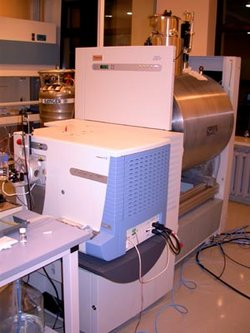The physics of FTICR is similar to that of a cyclotron
Cyclotron
In technology, a cyclotron is a type of particle accelerator. In physics, the cyclotron frequency or gyrofrequency is the frequency of a charged particle moving perpendicularly to the direction of a uniform magnetic field, i.e. a magnetic field of constant magnitude and direction...

at least in the first approximation.

In the simplest form (idealized) the relationship between the cyclotron frequency and the mass to charge ratio is given by: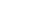where f = cyclotron frequency, q = ion charge, B = magnetic field strength and m = ion mass.

This is more often represented in angular frequency
Angular frequency
In physics, angular frequency ω is a scalar measure of rotation rate. Angular frequency is the magnitude of the vector quantity angular velocity...

: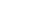where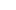is the angular cyclotron frequency which is related to frequency by the definition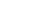.

Because of the quadrupolar electrical field used to trap the ions in the axial direction this relationship is only approximate. The axial electrical trapping results in axial oscillations within the trap with the (angular) frequency: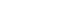Whereis a constant similar to the spring constant of a harmonic oscillator
Harmonic oscillator
In classical mechanics, a harmonic oscillator is a system that, when displaced from its equilibrium position, experiences a restoring force, F, proportional to the displacement, x: \vec F = -k \vec x \, where k is a positive constant....

and is dependent on voltage and the trap dimensions and geometry.

The electric field and the resulting axial harmonic motion reduces the cyclotron frequency and introduces a second radial motion called magnetron motion that occurs at the magnetron frequency. The cyclotron motion is still the frequency being used but the relationship above is not exact due to this phenomenon. The natural angular frequencies of motion are: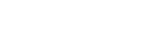where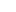is the axial trapping frequency due the axial electrical trapping and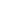is the reduced cyclotron (angular) frequency and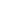is the magnetron (angular) frequency. Againis what is typically measured in FTICR. The meaning of this equation can be understood qualitatively by considering the case whereis small, which is generally true. In that case the value of the radical is just slightly less than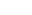and the value ofis just slightly less than(the cyclotron frequency has been slightly reduced). Forthe value of the radical is the same (slightly less than) but it is being subtracted fromresulting in a small number equal to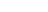(i.e. the exact amount that the cyclotron frequency was reduced by).

## ICR cell types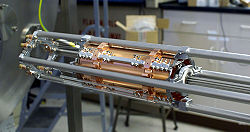A review of different cell geometries with their specific electric configurations is available in the literature. However, ICR cells can belong to one of the following two categories.

### Closed cells

Several closed ICR cells with different geometries were fabricated and their performance has been characterized. Grids were used as end caps to apply an axial electric field for trapping ions axially (parallel to the magnetic field lines). Ions can be either generated inside the cell (by Electron impact ionization) or can be injected to the cell from an external ionization source (such as Electrospray or MALDI). Nested ICR cells with double pair of grids were also fabricated to trap both positive and negative ions simultaneously.

### Open cells

The most common geometry is a cylinder, which is axially segmented into different parts to produce different ring electrodes. The central ring electrode is generally used for applying radial excitation electric field and detection. DC electric voltage is applied on the terminal ring electrodes to trap ions along the magnetic field lines. Open cylindrical cells with ring electrodes of different diameters have also been designed. They proved not only capable in trapping and detecting both ion polarities simultaneously, but also they succeeded to separate positive from negative ions radially. This presented a large discrimination in kinetic ion acceleration between positive and negative ions trapped simultaneously inside the new cell. Several ion axial acceleration schemes were recently written for ion-ion collision studies

## Stored waveform inverse Fourier transform

Stored waveform inverse Fourier transform (SWIFT) is a method for the creation of excitation waveforms for FTMS. The time-domain excitation waveform is formed from the inverse Fourier transform of the appropriate frequency-domain excitation spectrum, which is chosen to excite the resonance frequencies of selected ions. The SWIFT procedure can be used to select ions for tandem mass spectrometry
Tandem mass spectrometry
Tandem mass spectrometry, also known as MS/MS or MS2, involves multiple steps of mass spectrometry selection, with some form of fragmentation occurring in between the stages.-Tandem MS instruments:...

experiments.